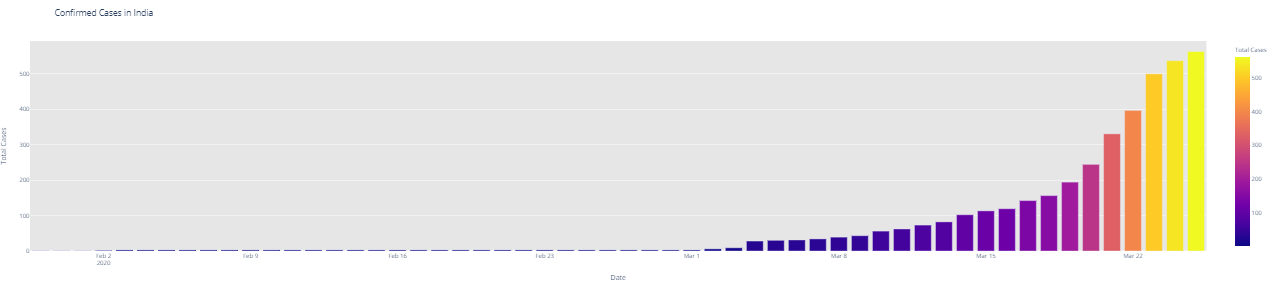## Predicting the Outbreak of COVID-19 Pandemic using Machine Learning

• Post author:

We are also going to highlight our data according to its geographical location in India.

Next, you’re going to use Plotly to obtain graphs depicting the trends of the rise of coronavirus cases across India.

```#This cell's code is required when you are working with plotly on colab
import plotly
plotly.io.renderers.default = 'colab'```
```# Rise of COVID-19 cases in India
fig = go.Figure()
fig.add_trace(go.Scatter(x=dbd_India['Date'], y = dbd_India['Total Cases'], mode='lines+markers',name='Total Cases'))
fig.update_layout(title_text='Trend of Coronavirus Cases in India (Cumulative cases)',plot_bgcolor='rgb(230, 230, 230)')
fig.show()```
```import plotly.express as px
fig = px.bar(dbd_India, x="Date", y="New Cases", barmode='group', height=400)
fig.update_layout(title_text='Coronavirus Cases in India on daily basis',plot_bgcolor='rgb(230, 230, 230)')
fig.show()```

### Part 2: Is the trend Similar to Italy, Wuhan & South Korea?

At this point, India had already crossed 500 cases. It still is very important to contain the situation in the coming days. The numbers of coronavirus patients had started doubling after many countries hit the 100 marks, and almost starting increasing exponentially.

```# import plotly.express as px
fig = px.bar(dbd_India, x="Date", y="Total Cases", color='Total Cases', orientation='v', height=600,
title='Confirmed Cases in India', color_discrete_sequence = px.colors.cyclical.IceFire) '''Colour Scale for plotly
https://plot.ly/python/builtin-colorscales/ '''
fig.update_layout(plot_bgcolor='rgb(230, 230, 230)')
fig.show()
fig = px.bar(dbd_Italy, x="Date", y="Total Cases", color='Total Cases', orientation='v', height=600,
title='Confirmed Cases in Italy', color_discrete_sequence = px.colors.cyclical.IceFire)
fig.update_layout(plot_bgcolor='rgb(230, 230, 230)')
fig.show()
fig = px.bar(dbd_Korea, x="Date", y="Total Cases", color='Total Cases', orientation='v', height=600,
title='Confirmed Cases in South Korea', color_discrete_sequence = px.colors.cyclical.IceFire)
fig.update_layout(plot_bgcolor='rgb(230, 230, 230)')
fig.show()
fig = px.bar(dbd_Wuhan, x="Date", y="Total Cases", color='Total Cases', orientation='v', height=600,
title='Confirmed Cases in Wuhan', color_discrete_sequence = px.colors.cyclical.IceFire)
fig.update_layout(plot_bgcolor='rgb(230, 230, 230)')
fig.show()```From the visualization above, one can infer the following:

• Confirmed cases in India is rising exponentially with no fixed pattern (Very less test in India)
• Confirmed cases in Italy is rising exponentially with a certain fixed pattern
• Confirmed cases in S.Korea is rising gradually
• There have been almost a negligible number confirmed cases in Wuhan a week.
```# import plotly.graph_objects as go
from plotly.subplots import make_subplots
fig = make_subplots(
rows=2, cols=2,
specs=[[{}, {}],
[{"colspan": 2}, None]],
subplot_titles=("S.Korea","Italy", "India","Wuhan"))
marker=dict(color=dbd_Korea['Total Cases'], coloraxis="coloraxis")),1, 1)
marker=dict(color=dbd_Italy['Total Cases'], coloraxis="coloraxis")),1, 2)
marker=dict(color=dbd_India['Total Cases'], coloraxis="coloraxis")),2, 1)
#                     marker=dict(color=dbd_Wuhan['Total Cases'], coloraxis="coloraxis")),2, 2)
fig.update_layout(coloraxis=dict(colorscale='Bluered_r'), showlegend=False,title_text="Total Confirmed cases(Cumulative)")
fig.update_layout(plot_bgcolor='rgb(230, 230, 230)')
fig.show()```
```# import plotly.graph_objects as go
title = 'Main Source for News'
labels = ['S.Korea', 'Italy', 'India']
colors = ['rgb(122,128,0)', 'rgb(255,0,0)', 'rgb(49,130,189)']
mode_size = [10, 10, 12]
line_size = [1, 1, 8]
fig = go.Figure()
y=dbd_Korea['Total Cases'],mode='lines',
name=labels,
line=dict(color=colors, width=line_size),
connectgaps=True))
y=dbd_Italy['Total Cases'],mode='lines',
name=labels,
line=dict(color=colors, width=line_size),
connectgaps=True))
y=dbd_India['Total Cases'],mode='lines',
name=labels,
line=dict(color=colors, width=line_size),
connectgaps=True))
annotations = []
annotations.append(dict(xref='paper', yref='paper', x=0.5, y=-0.1,
xanchor='center', yanchor='top',
text='Days after crossing 100 cases ',
font=dict(family='Arial',
size=12,
color='rgb(150,150,150)'),
showarrow=False))
fig.update_layout(annotations=annotations,plot_bgcolor='white',yaxis_title='Cumulative cases')
fig.show()```

### Part 3: Exploring Worldwide Data

The following code will give you tabular data about the location and status of confirmed cases by date.

```df = pd.read_csv('/content/covid_19_clean_complete.csv',parse_dates=['Date'])
df.rename(columns={'ObservationDate':'Date', 'Country/Region':'Country'}, inplace=True)
df_confirmed.rename(columns={'Country/Region':'Country'}, inplace=True)
df_recovered.rename(columns={'Country/Region':'Country'}, inplace=True)
df_deaths.rename(columns={'Country/Region':'Country'}, inplace=True)
```df2 = df.groupby(["Date", "Country", "Province/State"])[['Date', 'Province/State', 'Country', 'Confirmed', 'Deaths', 'Recovered']].sum().reset_index()
```#Overall worldwide Confirmed/ Deaths/ Recovered cases
```confirmed = df.groupby('Date').sum()['Confirmed'].reset_index()
deaths = df.groupby('Date').sum()['Deaths'].reset_index()
recovered = df.groupby('Date').sum()['Recovered'].reset_index()```
```fig = go.Figure()
#Plotting datewise confirmed cases
fig.add_trace(go.Scatter(x=deaths['Date'], y=deaths['Deaths'], mode='lines+markers', name='Deaths', line=dict(color='Red', width=2)))
fig.add_trace(go.Scatter(x=recovered['Date'], y=recovered['Recovered'], mode='lines+markers', name='Recovered', line=dict(color='Green', width=2)))
fig.update_layout(title='Worldwide NCOVID-19 Cases', xaxis_tickfont_size=14,yaxis=dict(title='Number of Cases'))
fig.show()```

### Part 4: Forecasting Total Number of Cases Worldwide

In this segment, we’re going to generate a week ahead forecast of confirmed cases of COVID-19 using Prophet, with specific prediction intervals by creating a base model both with and without tweaking of seasonality-related parameters and additional regressors.

Prophet is open source software released by Facebook’s Core Data Science team. It is available for download on CRAN and PyPI.

We use Prophet, a procedure for forecasting time series data based on an additive model where non-linear trends are fit with yearly, weekly, and daily seasonality, plus holiday effects. It works best with time series that have strong seasonal effects and several seasons of historical data. Prophet is robust to missing data and shifts in the trend, and typically handles outliers well.

#### Why Prophet?

• Accurate and fast: Prophet is used in many applications across Facebook for producing reliable forecasts for planning and goal setting. Facebook finds it to perform better than any other approach in the majority of cases. It fit models in Stan, so that you get forecasts in just a few seconds.

• Fully automatic: Get a reasonable forecast on messy data with no manual effort. Prophet is robust to outliers, missing data, and dramatic changes in your time series.

• Tunable forecasts: The Prophet procedure includes many possibilities for users to tweak and adjust forecasts. You can use human-interpretable parameters to improve your forecast by adding your domain knowledge

• Available in R or Python: Facebook has implemented the Prophet procedure in R and Python. Both of them share the same underlying Stan code for fitting. You can use whatever language you’re comfortable with to get forecasts.

```from fbprophet import Prophet
confirmed = df.groupby('Date').sum()['Confirmed'].reset_index()
deaths = df.groupby('Date').sum()['Deaths'].reset_index()
recovered = df.groupby('Date').sum()['Recovered'].reset_index()```

The input to Prophet is always a data frame with two columns: ds and y. The ds (datestamp) column should be of a format expected by Pandas, ideally YYYY-MM-DD for a date or YYYY-MM-DD HH:MM:SS for a timestamp. The y column must be numeric and represents the measurement we wish to forecast.

```confirmed.columns = ['ds','y']
#confirmed['ds'] = confirmed['ds'].dt.date
confirmed['ds'] = pd.to_datetime(confirmed['ds'])
confirmed.tail()```

Generating a week ahead forecast of confirmed cases of COVID-19 using Prophet, with a 95% prediction interval by creating a base model with no tweaking of seasonality-related parameters and additional regressors.

`m = Prophet(interval_width=0.95) m.fit(confirmed) future = m.make_future_dataframe(periods=7) future.tail()`

The predict method will assign each row in future a predicted value which it names yhat. If you pass on historical dates, it will provide an in-sample fit. The forecast object here is a new data-frame that includes a column yhat with the forecast, as well as columns for components and uncertainty intervals.